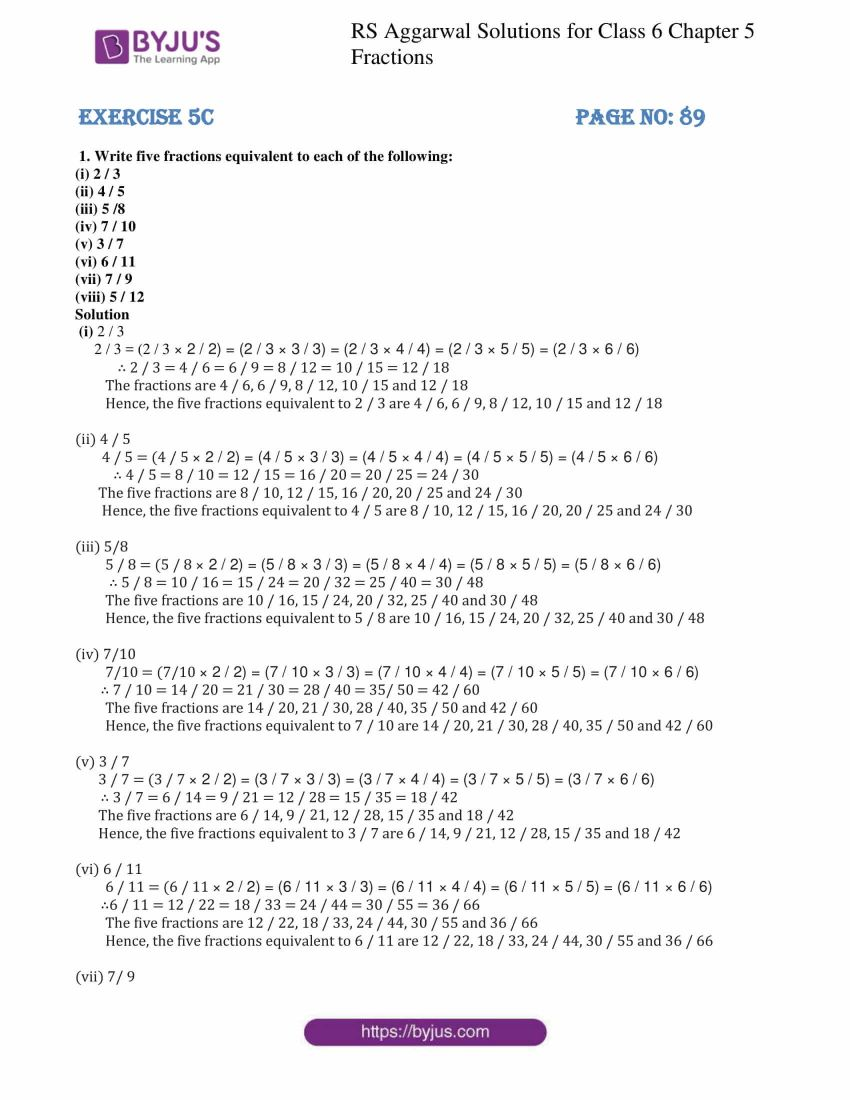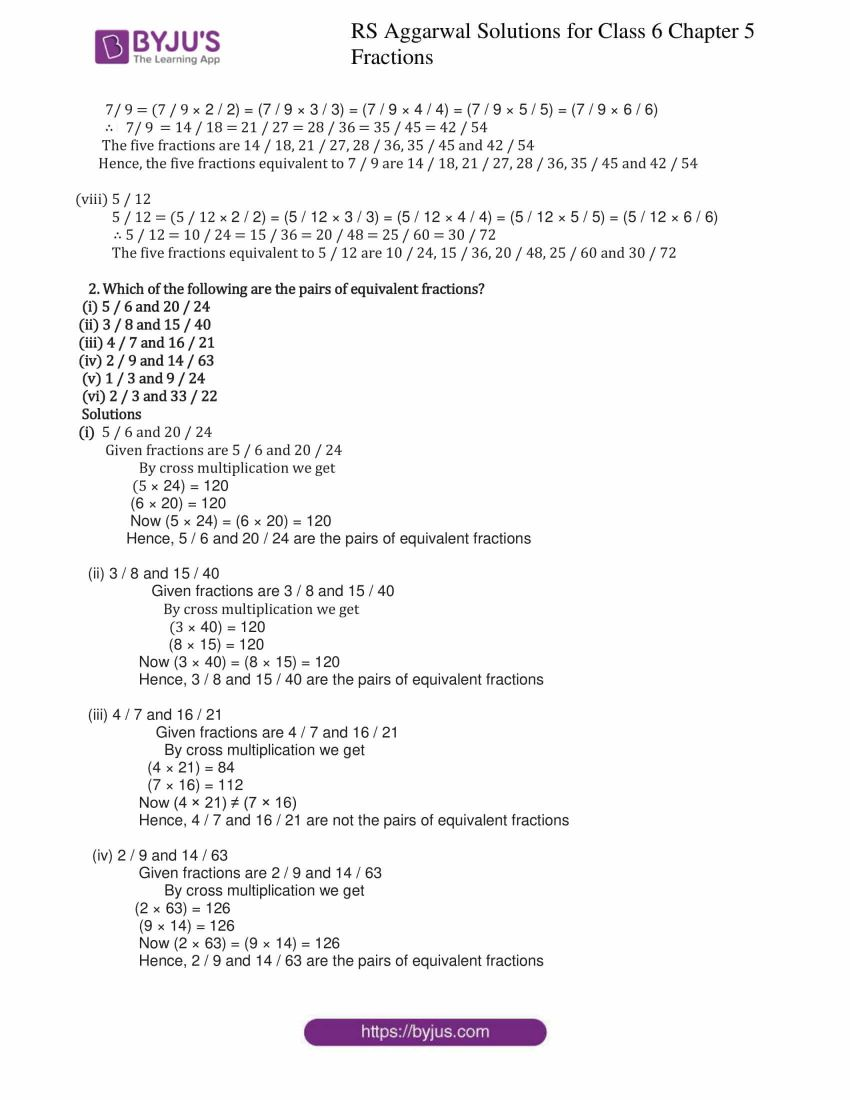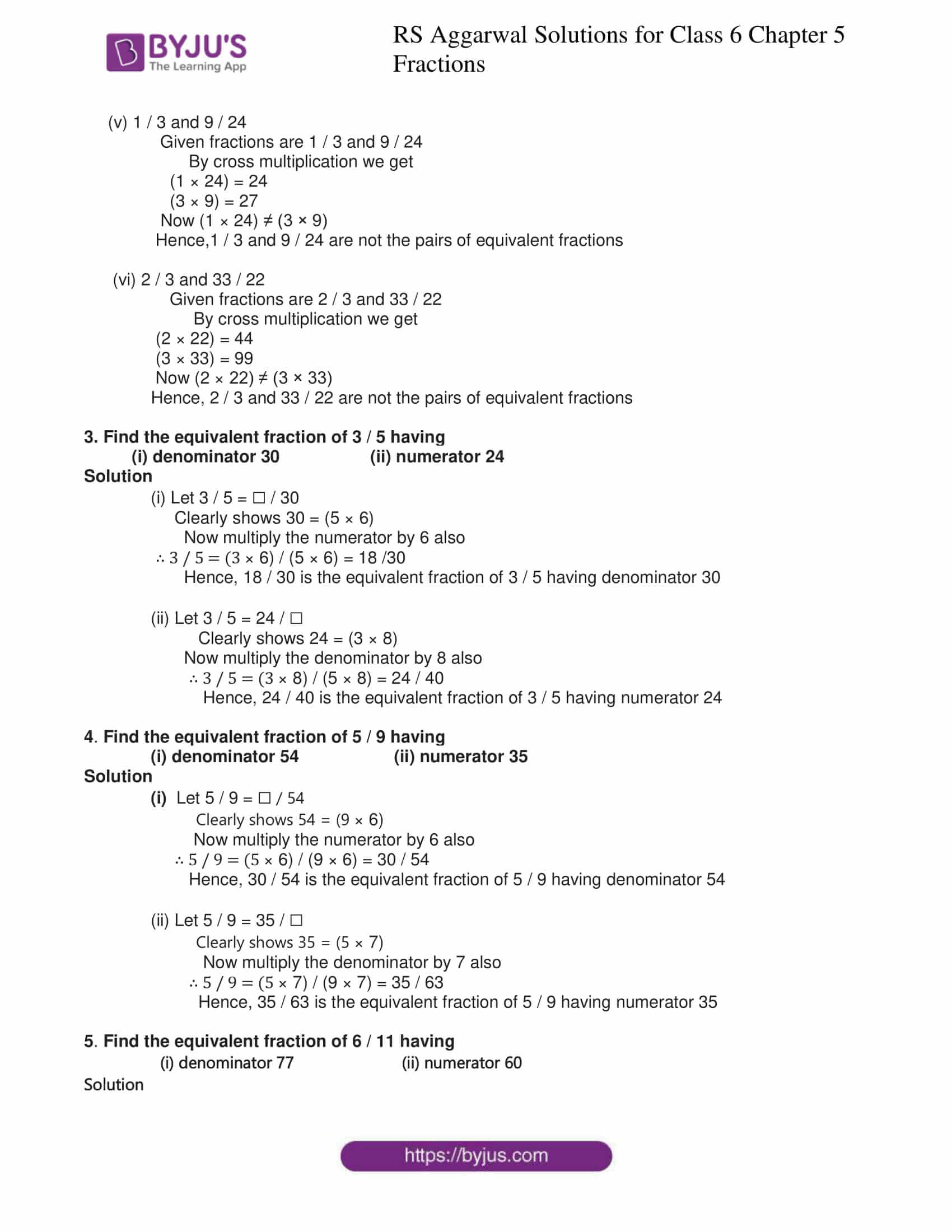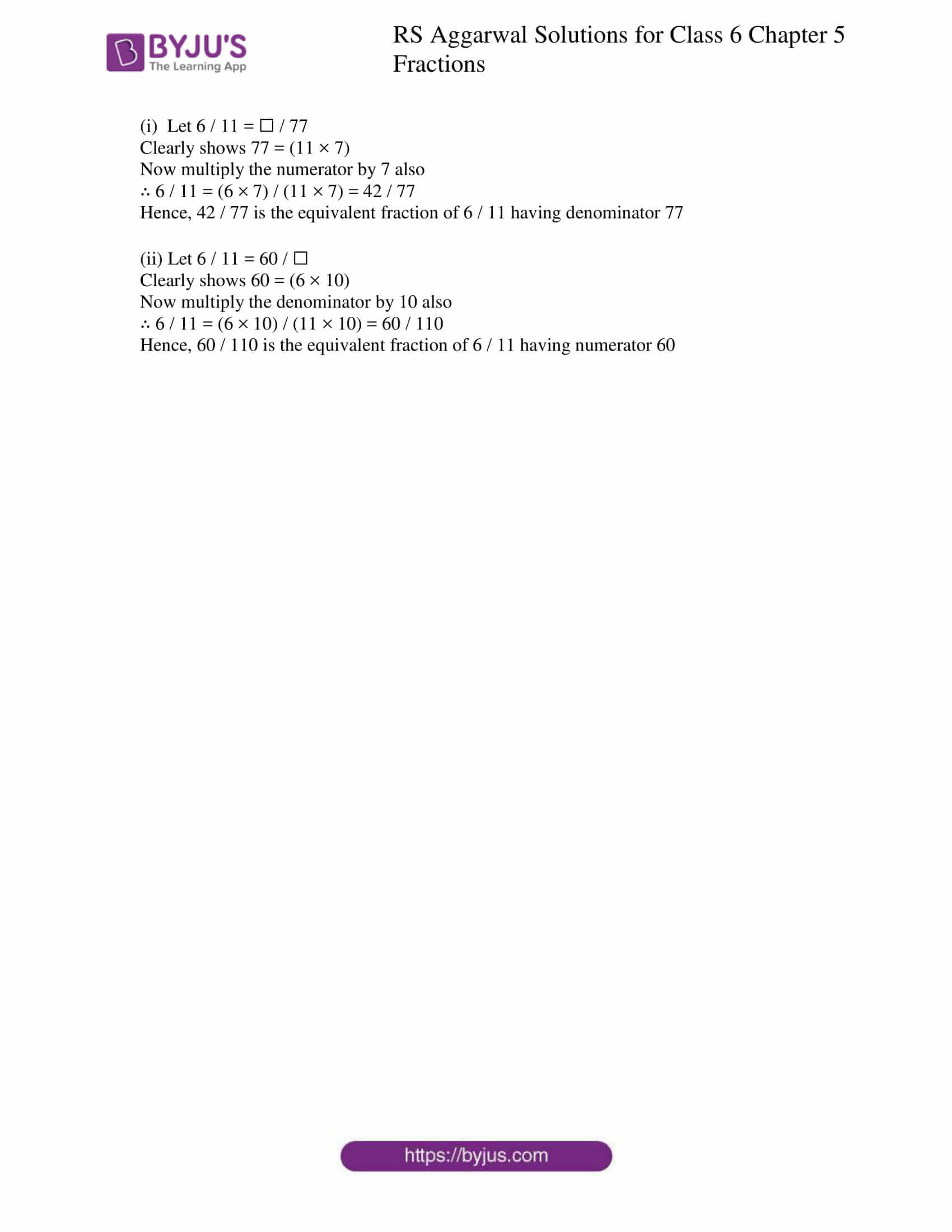# RS Aggarwal Solutions for Class 6 Maths Chapter 5 Fractions Exercise 5C

RS Aggarwal Solutions for Class 6 Maths Chapter 5 Fractions Exercise 5C are in PDF form for students to refer to. Exercise 5C of RS Aggarwal Solutions has questions based on concepts like Equivalent Fractions. Two or more fractions representing the same part of a whole are called equivalent fractions. The solutions are designed by experts in a comprehensive manner to make it easy for students while studying. Download PDF of solutions in the respective links given below.

## Download PDF of RS Aggarwal Solutions for Class 6 Maths Chapter 5 Fractions Exercise 5C### Access answers to Maths RS Aggarwal Solutions for Class 6 Chapter 5 Fractions Exercise 5C

1. Write five fractions equivalent to each of the following:

(i) 2 / 3

(ii) 4 / 5

(iii) 5 /8

(iv) 7 / 10

(v) 3 / 7

(vi) 6 / 11

(vii) 7 / 9

(viii) 5 / 12

Solution

(i) 2 / 3

2 / 3 = (2 / 3 × 2 / 2) = (2 / 3 × 3 / 3) = (2 / 3 × 4 / 4) = (2 / 3 × 5 / 5) = (2 / 3 × 6 / 6)

∴ 2 / 3 = 4 / 6 = 6 / 9 = 8 / 12 = 10 / 15 = 12 / 18

The fractions are 4 / 6, 6 / 9, 8 / 12, 10 / 15 and 12 / 18

Hence, the five fractions equivalent to 2 / 3 are 4 / 6, 6 / 9, 8 / 12, 10 / 15 and 12 / 18

(ii) 4 / 5

4 / 5 = (4 / 5 × 2 / 2) = (4 / 5 × 3 / 3) = (4 / 5 × 4 / 4) = (4 / 5 × 5 / 5) = (4 / 5 × 6 / 6)

∴ 4 / 5 = 8 / 10 = 12 / 15 = 16 / 20 = 20 / 25 = 24 / 30

The five fractions are 8 / 10, 12 / 15, 16 / 20, 20 / 25 and 24 / 30

Hence, the five fractions equivalent to 4 / 5 are 8 / 10, 12 / 15, 16 / 20, 20 / 25 and 24 / 30

(iii) 5/8

5 / 8 = (5 / 8 × 2 / 2) = (5 / 8 × 3 / 3) = (5 / 8 × 4 / 4) = (5 / 8 × 5 / 5) = (5 / 8 × 6 / 6)

∴ 5 / 8 = 10 / 16 = 15 / 24 = 20 / 32 = 25 / 40 = 30 / 48

The five fractions are 10 / 16, 15 / 24, 20 / 32, 25 / 40 and 30 / 48

Hence, the five fractions equivalent to 5 / 8 are 10 / 16, 15 / 24, 20 / 32, 25 / 40 and 30 / 48

(iv) 7/10

7/10 = (7/10 × 2 / 2) = (7 / 10 × 3 / 3) = (7 / 10 × 4 / 4) = (7 / 10 × 5 / 5) = (7 / 10 × 6 / 6)

∴ 7 / 10 = 14 / 20 = 21 / 30 = 28 / 40 = 35/ 50 = 42 / 60

The five fractions are 14 / 20, 21 / 30, 28 / 40, 35 / 50 and 42 / 60

Hence, the five fractions equivalent to 7 / 10 are 14 / 20, 21 / 30, 28 / 40, 35 / 50 and 42 / 60

(v) 3 / 7

3 / 7 = (3 / 7 × 2 / 2) = (3 / 7 × 3 / 3) = (3 / 7 × 4 / 4) = (3 / 7 × 5 / 5) = (3 / 7 × 6 / 6)

∴ 3 / 7 = 6 / 14 = 9 / 21 = 12 / 28 = 15 / 35 = 18 / 42

The five fractions are 6 / 14, 9 / 21, 12 / 28, 15 / 35 and 18 / 42

Hence, the five fractions equivalent to 3 / 7 are 6 / 14, 9 / 21, 12 / 28, 15 / 35 and 18 / 42

(vi) 6 / 11

6 / 11 = (6 / 11 × 2 / 2) = (6 / 11 × 3 / 3) = (6 / 11 × 4 / 4) = (6 / 11 × 5 / 5) = (6 / 11 × 6 / 6)

∴6 / 11 = 12 / 22 = 18 / 33 = 24 / 44 = 30 / 55 = 36 / 66

The five fractions are 12 / 22, 18 / 33, 24 / 44, 30 / 55 and 36 / 66

Hence, the five fractions equivalent to 6 / 11 are 12 / 22, 18 / 33, 24 / 44, 30 / 55 and 36 / 66

(vii) 7/ 9

7/ 9 = (7 / 9 × 2 / 2) = (7 / 9 × 3 / 3) = (7 / 9 × 4 / 4) = (7 / 9 × 5 / 5) = (7 / 9 × 6 / 6)

∴ 7/9 = 14 / 18 = 21 / 27 = 28 / 36 = 35 / 45 = 42 / 54

The five fractions are 14 / 18, 21 / 27, 28 / 36, 35 / 45 and 42 / 54

Hence, the five fractions equivalent to 7 / 9 are 14 / 18, 21 / 27, 28 / 36, 35 / 45 and 42 / 54

(viii) 5 / 12

5 / 12 = (5 / 12 × 2 / 2)

= (5 / 12 × 3 / 3)

= (5 / 12 × 4 / 4)

= (5 / 12 × 5 / 5)

= (5 / 12 × 6 / 6)

∴ 5 / 12

= 10 / 24

= 15 / 36

= 20 / 48

= 25 / 60

= 30 / 72

The five fractions equivalent to 5 / 12 are 10 / 24, 15 / 36, 20 / 48, 25 / 60 and 30 / 72

2. Which of the following are the pairs of equivalent fractions?

(i) 5 / 6 and 20 / 24

(ii) 3 / 8 and 15 / 40

(iii) 4 / 7 and 16 / 21

(iv) 2 / 9 and 14 / 63

(v) 1 / 3 and 9 / 24

(vi) 2 / 3 and 33 / 22

Solutions

(i) 5 / 6 and 20 / 24

Given fractions are 5 / 6 and 20 / 24

By cross multiplication we get

(5 × 24) = 120

(6 × 20) = 120

Now (5 × 24) = (6 × 20) = 120

Hence, 5 / 6 and 20 / 24 are the pairs of equivalent fractions

(ii) 3 / 8 and 15 / 40

Given fractions are 3 / 8 and 15 / 40

By cross multiplication we get

(3 × 40) = 120

(8 × 15) = 120

Now (3 × 40) = (8 × 15) = 120

Hence, 3 / 8 and 15 / 40 are the pairs of equivalent fractions

(iii) 4 / 7 and 16 / 21

Given fractions are 4 / 7 and 16 / 21

By cross multiplication we get

(4 × 21) = 84

(7 × 16) = 112

Now (4 × 21) ≠ (7 × 16)

Hence, 4 / 7 and 16 / 21 are not the pairs of equivalent fractions

(iv) 2 / 9 and 14 / 63

Given fractions are 2 / 9 and 14 / 63

By cross multiplication we get

(2 × 63) = 126

(9 × 14) = 126

Now (2 × 63) = (9 × 14) = 126

Hence, 2 / 9 and 14 / 63 are the pairs of equivalent fractions

(v) 1 / 3 and 9 / 24

Given fractions are 1 / 3 and 9 / 24

By cross multiplication we get

(1 × 24) = 24

(3 × 9) = 27

Now (1 × 24) ≠ (3 × 9)

Hence,1 / 3 and 9 / 24 are not the pairs of equivalent fractions

(vi) 2 / 3 and 33 / 22

Given fractions are 2 / 3 and 33 / 22

By cross multiplication we get

(2 × 22) = 44

(3 × 33) = 99

Now (2 × 22) ≠ (3 × 33)

Hence, 2 / 3 and 33 / 22 are not the pairs of equivalent fractions

3. Find the equivalent fraction of 3 / 5 having

(i) denominator 30 (ii) numerator 24

Solution

(i) Let 3 / 5 = ☐ / 30

Clearly shows 30 = (5 × 6)

Now multiply the numerator by 6 also

∴ 3 / 5 = (3 × 6) / (5 × 6) = 18 /30

Hence, 18 / 30 is the equivalent fraction of 3 / 5 having denominator 30

(ii) Let 3 / 5 = 24 / ☐

Clearly shows 24 = (3 × 8)

Now multiply the denominator by 8 also

∴ 3 / 5 = (3 × 8) / (5 × 8) = 24 / 40

Hence, 24 / 40 is the equivalent fraction of 3 / 5 having numerator 24

4. Find the equivalent fraction of 5 / 9 having

(i) denominator 54 (ii) numerator 35

Solution

(i) Let 5 / 9 = ☐ / 54

Clearly shows 54 = (9 × 6)

Now multiply the numerator by 6 also

∴ 5 / 9 = (5 × 6) / (9 × 6) = 30 / 54

Hence, 30 / 54 is the equivalent fraction of 5 / 9 having denominator 54

(ii) Let 5 / 9 = 35 / ☐

Clearly shows 35 = (5 × 7)

Now multiply the denominator by 7 also

∴ 5 / 9 = (5 × 7) / (9 × 7) = 35 / 63

Hence, 35 / 63 is the equivalent fraction of 5 / 9 having numerator 35

5. Find the equivalent fraction of 6 / 11 having

(i) denominator 77 (ii) numerator 60

Solution

(i) Let 6 / 11 = ☐ / 77

Clearly shows 77 = (11 × 7)

Now multiply the numerator by 7 also

∴ 6 / 11 = (6 × 7) / (11 × 7) = 42 / 77

Hence, 42 / 77 is the equivalent fraction of 6 / 11 having denominator 77

(ii) Let 6 / 11 = 60 / ☐

Clearly shows 60 = (6 × 10)

Now multiply the denominator by 10 also

∴ 6 / 11 = (6 × 10) / (11 × 10) = 60 / 110

Hence, 60 / 110 is the equivalent fraction of 6 / 11 having numerator 60